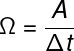Mechanics

# Kepler’s Second Law

Kepler’s second law , also known as the law of areas, states that the imaginary line connecting a planet to the Sun sweeps out equal areas in equal intervals of time. According to this law, the area covered by the radius vector that connects a planet to the Sun during a time interval is constant and is called the areolar velocity .

## Introduction to Kepler’s second law

Kepler ‘s second law follows directly from the principle of conservation of angular momentum . Angular momentum is the amount of motion related to rotating bodies , such as planets moving around the Sun. Angular momentum is a vector quantity , and its magnitude depends directly on the radius of the orbit and the speed with which the body moves. Thus, if the distance between the Sun and the planet increases, its speed must decrease and vice versa.

Kepler’s second law was able to show that, when we draw a line that goes from a planet to the Sun, the area swept by this line along the orbit will always be equal for equal intervals of time , regardless of what the initial position is. of the planet. This is because the area covered is shaped like an arc whose length is related to the speed at which the planet is moving, but its sides are determined by the initial and final distances from the Sun.

The rate at which an area is traversed by the radius vector that connects the planet to the Sun for a given time interval is known as areolar velocity , moreover, when a planet is in orbit around the Sun, this velocity is always constant , check:Ω – areolar velocity (m²/s)

A – area (m²)

Δt – time interval (s)

It is important to note that the areolar velocity is different from the orbital velocity of the planet. The latter changes according to the distance between the planet and the Sun — near perihelion , the orbital speed increases, and at aphelion , it decreases, thanks to variations in the magnitude of gravitational attraction.

## Kepler’s second law summary

According to Kepler’s second law:

• The line connecting a planet to the Sun covers equal areas in equal intervals of time.
• The rate at which the area is traversed by the ray vector is known as the areolar velocity.

## Exercises solved on Kepler’s second law

Question 1) (UFRGS) The ellipse, in the figure below, represents the orbit of a planet around a star S. The points along the ellipse represent successive positions of the planet, separated by equal time intervals. The alternately colored regions represent the areas swept by the radius of the trajectory in these time intervals. In the figure, in which the dimensions of the stars and the size of the orbit are not to scale, the line segment SH represents the focal ray of the point H of length p.

Considering that the only force acting on the star-planet system is the gravitational force, the following statements are made:

I. The areas S 1 and S 2 , swept by the radius of the trajectory, are equal.

II. The orbit period is proportional to ap 3 .

III. The tangential velocities of the planet at points A and H, V A and V H are such that V A > V H .

Which ones are correct?

a) only I

b) Only I and II

c) Only I and III

d) Only II and III

e) I, II and III

Template: Letter C

Resolution:

Let’s analyze the alternatives:

I – TRUE

II – FALSE. The square of the orbit’s period is proportional to the cube of the mean radius, according to Kepler’s 3rd law.

III – TRUE

Question 2) (Acafe) Astronomers have found an exoplanet (a planet orbiting a star other than the Sun) with an eccentricity much greater than normal. The eccentricity reveals how elongated its orbit around its star is. In the case of Earth, the eccentricity is 0.017, much smaller than the value of 0.96 for that planet, which was called HD 20782.

In the following figures, the orbits of Earth and HD 20782 can be compared.

In this sense, mark the correct alternative:

a) Kepler’s laws do not apply to HD 20782 because its orbit is not circular like Earth’s.

b) Newton’s laws of gravitation do not apply to HD 20782 because its orbit is too eccentric.

c) The gravitational force between the planet HD 20782 and its star is maximum when it is passing aphelion.

d) Planet HD 20782 has an accelerated motion when moving from aphelion to perihelion.

Template : Letter D

Resolution:

At perihelion, the planet is at the shortest distance to the Sun, so it is subject to the greatest gravitational pull that its orbit allows, so the correct answer is the letter D.

Question 3) (UFSM) Advances in observational techniques have allowed astronomers to track an increasing number of celestial objects orbiting the Sun. The figure shows, on an arbitrary scale, the orbits of Earth and a comet (body sizes are not to scale). Based on the figure, analyze the statements:

I. Given the large difference between the masses of the Sun and the comet, the gravitational attraction exerted by the comet on the Sun is much smaller than the attraction exerted by the Sun on the comet.

II. The comet’s velocity is constant at all points in the orbit.

III. The comet’s translation period is longer than one Earth year.

Is(are) correct:

a) only I

b) only III

c) only I and II

d) only II and III

e) I, II and III

Template: letter B

Resolution :

Let’s analyze the alternatives:

I – FALSE. According to Newton’s third law , these forces must have equal magnitudes.

II – FALSE. In elliptical paths, the motion is accelerated when the star approaches the Sun.

III – TRUE